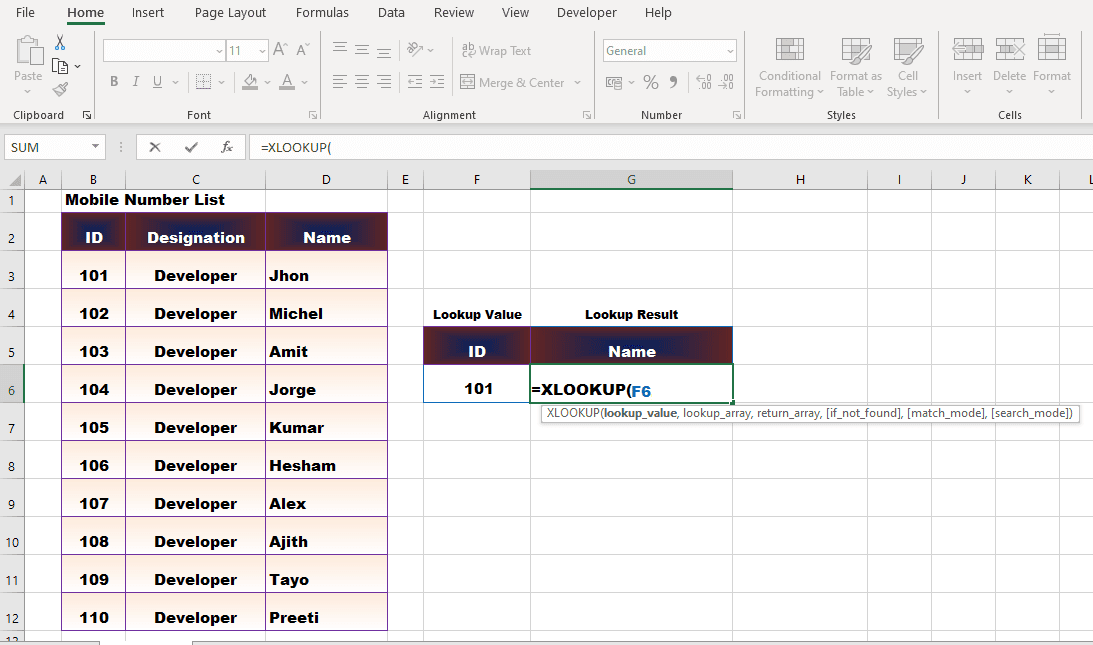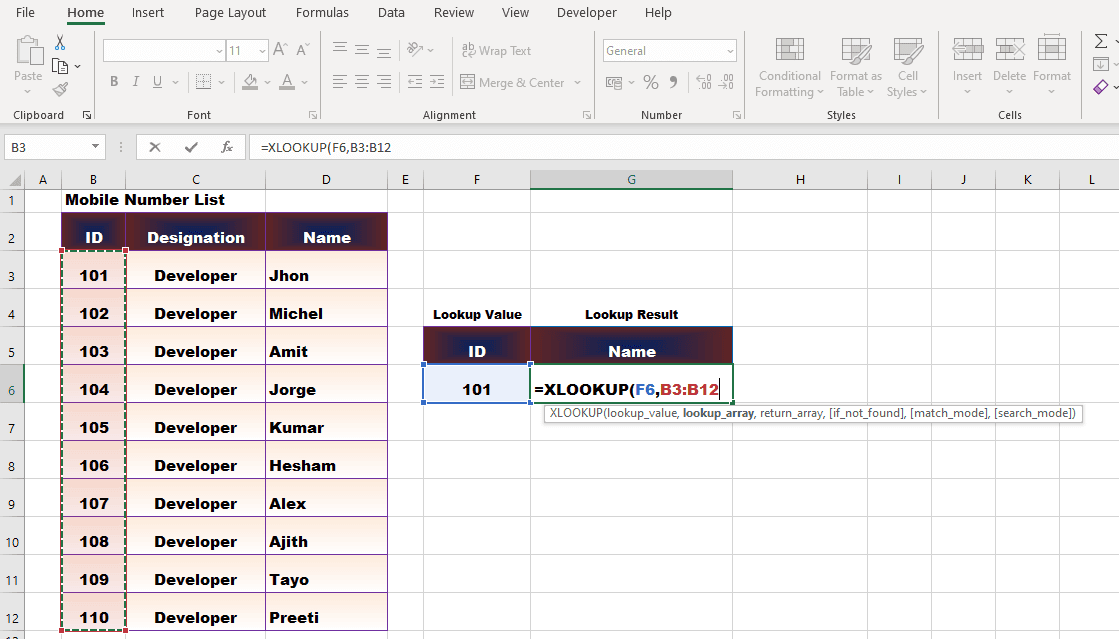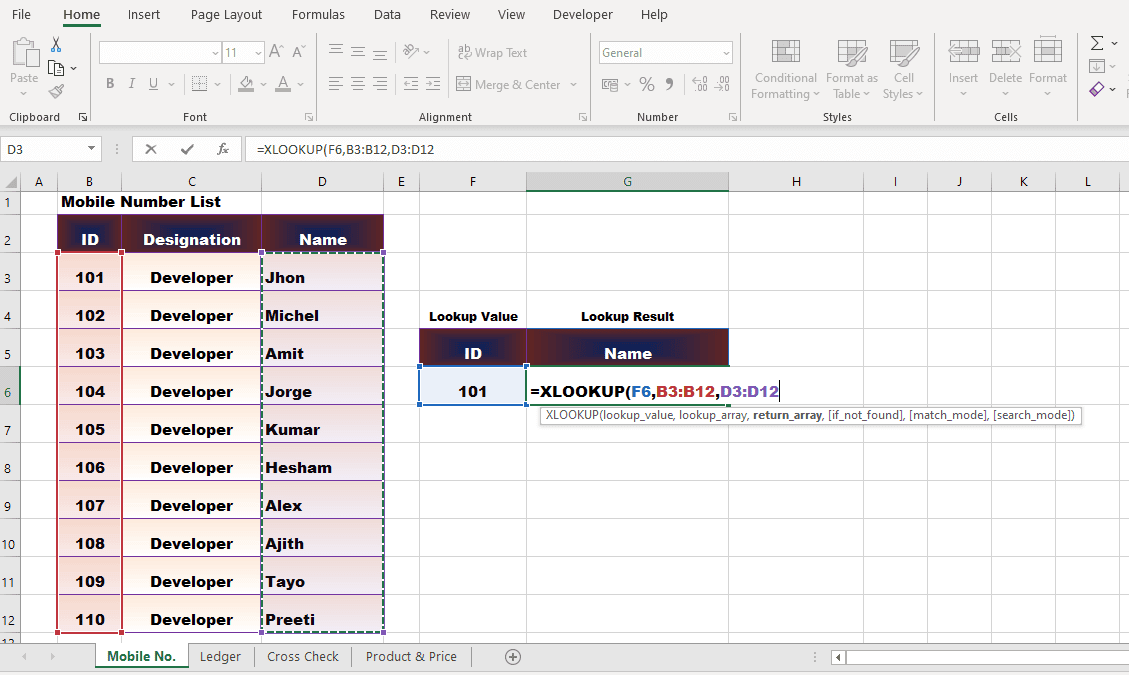Excel's new XLOOKUP function is now generally available. Learn How to Apply XLOOKUP function? - KING OF EXCEL

## Friday, March 6, 2020

Excel's new XLOOKUP function is now generally available.Here comes the Xlookup function. Now say goodbye to the Vlookup function. How to Apply Xlookup Function Difference Between Xlookup Function and Vlookup Function

## Here comes the Xlookup function. Now say goodbye to the Vlookup function.

The most popular function of Excel is called Vlookup Function. A few months ago Microsoft launched a new function Xlookup Function.Now it has been added in the update to Office 365. In this article, let's learn how to Apply XLOOKUP Function and what is different from Vlookup Function.

## How to Apply XLOOKUP function?

Xlookup Function is very easy to Use. The same process as the Vlookup Function. The result will also show the same way. As per Employee ID, we have to show the name of Employee with this function. We have a data Employee ID, Designation and Name. Now we will apply Xlookup Function according to ID.

Lookup_Value - In the cell applying the formula type =VLOOKUP(Lookup_Value will be highlighted as you type the bracket. The lookup Value is the code number. Click the cell of the code number. The criterion from which we want to display the result will be the criterion lookup value.

= XLOOKUP(F6Lookup_array - Type commas. After Lookup_array is highlighted, select the ID range from the data. Lookup value range has to be selected in the data. As we are searching results by ID, the entire column of the ID will be Lookup_array or Lookup Range.

=XLOOKUP(F6,B3:B12return_array - type comma again. Select the range of name as soon as return_array is highlighted. The column from which we will get the result will be the column return_array.

=XLOOKUP(F6, B3:B12,D3:D12)IF_Not_Found - N/A will be displayed when typing the ID in Different to the range. Instead of this error, you can show any text, type a comma after Return_array, if the IF_Not_Found is highlighted, write the text in double inverted comma. E.g. "Not Found".On pressing enter, you will get the result of Xlookup Function.Result of additional ID over range.What is the difference between Xlookup Function and Vlookup Function?

1. In Vlookup Function, we had to select the data and give the number of columns of Lookup Value from the Lookup Range range. There is no need to select complete data in Xlookup Function. Only Lookup Range and Result Range have to be selected.

2. If Lookup Value is out of range, then we had to hide the error by applying Iferror Function to remove the error. Xlooup Function now has the option of IF_Not_Found for error results.